# Texas Go Math Kindergarten Lesson 17.3 Answer Key Identify Rectangles

Refer to our Texas Go Math Kindergarten Answer Key Pdf to score good marks in the exams. Test yourself by practicing the problems from Texas Go Math Kindergarten Lesson 17.3 Answer Key Identify Rectangles.

## Texas Go Math Kindergarten Lesson 17.3 Answer Key Identify Rectangles

Essential Question
How can you identify and describe rectangles?

Explore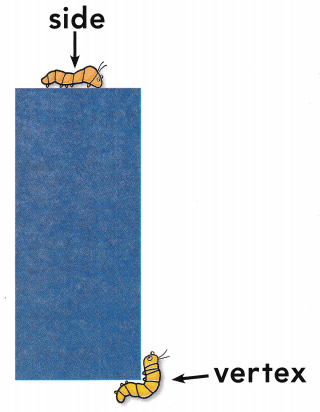Directions
Use your finger to trace around the rectangle. Talk about the number of sides and the number of square vertices, Draw an arrow pointing to ‘another square vertex. Use a pencil or crayon to trace around the rectangle.

Share and ShowQuestion 1.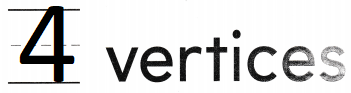Explanation:
A rectangle is a type of quadrilateral,
whose opposite sides are equal and parallel.
A rectangle has 4 vertices.

Question 2.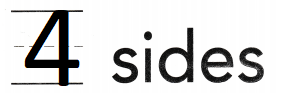Explanation:
A rectangle is a type of quadrilateral,
whose opposite sides are equal and parallel.
A rectangle has 4 sides.

Directions
1. Place a counter on each corner, or vertex. Write how many corners, or vertices. 2. Trace around the sides Write how many sides.

Question 3.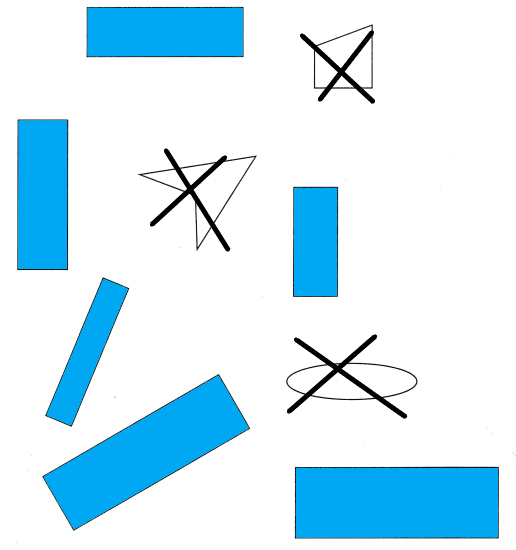Explanation:
All rectangles are colored in blue
and the shapes which are not rectangles crossed.

Directions
3. Sort the shapes. Use blue to color the rectangles. Mark an X on the shapes that are not rectangles.

Home Activity

• Have your child describe a rectangle.

Problem Solving

Question 4.Explanation:
A rectangle is a type of quadrilateral,
whose opposite sides are equal and parallel.
Rectangle has 4 sides and 4 vertices.

Question 5.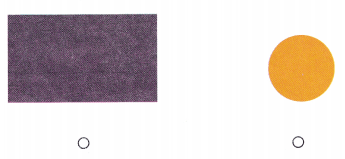Explanation:
A rectangle is a type of quadrilateral,
whose opposite sides are equal and parallel.
Rectangle has 4 sides and 4 vertices.

Directions
4. Draw a shape with 4 straight sides and 4 square vertices. Tell a friend the name of the shape. 5. Choose the correct answer. Which shape has four sides and four square vertices?

### Texas Go Math Kindergarten Lesson 17.3 Homework and Practice

Question 1.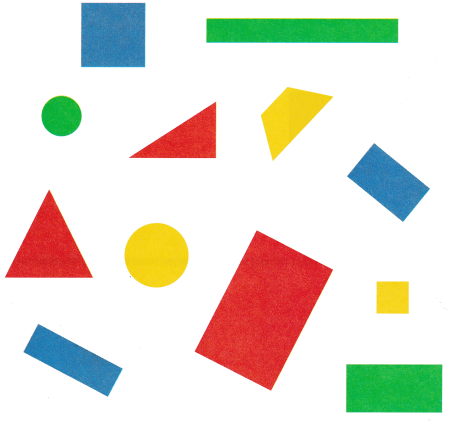Explanation:
A rectangle is a type of quadrilateral,
whose opposite sides are equal and parallel.
Rectangle has 4 sides and 4 vertices.

Directions
1. Sort the shapes by rectangles and not rectangles. Mark an X on all of the rectangles. Draw a line under all the shapes that are not rectangles.

Texas Test Prep

Lesson Check

Question 2.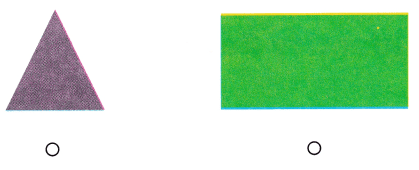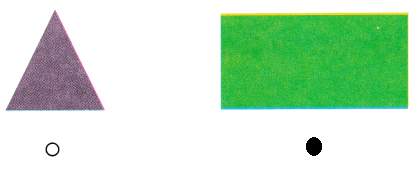Explanation:
A rectangle is a type of quadrilateral,
whose opposite sides are equal and parallel.
Rectangle has 4 sides and 4 vertices.

Question 3.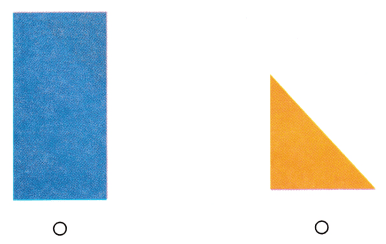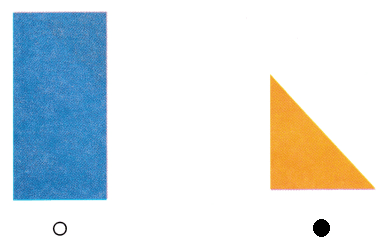Explanation:
A rectangle is a type of quadrilateral,
whose opposite sides are equal and parallel.
Rectangle has 4 sides and 4 vertices.

Question 4.